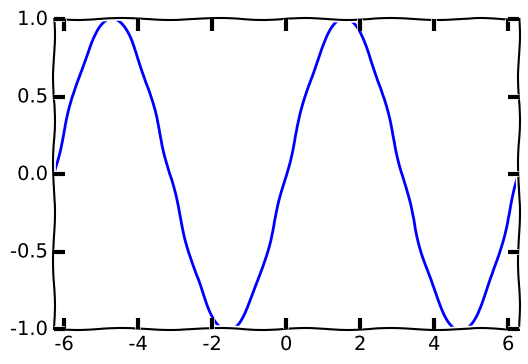# Homework Guidelines¶

Homework should always be done as a jupyter notebook, like this one. Problems not following guidelines will lose 25% if the issues are small (like sparsely commented code) or if serious (equations are in plain text, no work shown) the problem will not be graded and receive a 0. Read more about notebooks here. Here is an example of a well-constructed notebook. The following guidelines for homework apply:

• Work must be shown for any credit. Use common sense here. You don't need to write out very easy steps, but you also shouldn't be writing down answers to questions without any work shown. Refer to the homework solutions for examples.
• All writing should be done in markdown. Go here for a description of how to use Markdown

# Python Example¶

In :
#This is a python example

#First we enable plotting for the notbook. This statement is specific to notebooks
#and note a part of python.
%matplotlib inline

#Now we important the libraries we need.
#The as keyword allows us to shorten their names when used
#below.
import matplotlib.pyplot as plt
import numpy as np

#make our plot look fun and cartoony
plt.xkcd()

#The from keyword allows us to import only certain items
from math import pi

#Create a set of points from -2pi to 2pi spaced by 0.01 and calculate the sine of them
x = np.arange(-2*pi, 2*pi, 0.01) #notice that np refers to numpy due to the as statement.
y = np.sin(x)

#Make the plot and set x-limit
plt.plot(x,y)
plt.xlim(-2*pi, 2*pi)

#show the plot
plt.show()

/opt/conda/lib/python3.5/site-packages/matplotlib/font_manager.py:1288: UserWarning: findfont: Font family ['Humor Sans', 'Comic Sans MS'] not found. Falling back to Bitstream Vera Sans
(prop.get_family(), self.defaultFamily[fontext]))# Equation Example¶

This is a small equation: $\ln x^2$. This equation has sub/super scripts: $P_e(s) = b^i$ This one has a sum: $\sum_i^N f_i(s)$. This is a big equation:

$$\frac{\partial V(\vec{s})}{\partial s_j} = \frac{\frac{\partial f(\vec{s})}{\partial s_j} - \frac{f(\vec{s})}{g_j(s_j)}\frac{\partial g_j(s_j)}{\partial s_j}}{\prod_i^N g(\vec{s})}$$
This is a small equation: $\ln x^2$. This equation has sub/super scripts: $P_e(s) = b^i$ This one has a sum: $\sum_i^N f_i(s)$. This is a big equation: $$\frac{\partial V(\vec{s})}{\partial s_j} = \frac{\frac{\partial f(\vec{s})}{\partial s_j} - \frac{f(\vec{s})}{g_j(s_j)}\frac{\partial g_j(s_j)}{\partial s_j}}{\prod_i^N g(\vec{s})}$$